Anúncio

# W1_EE566.pptx

28 de Mar de 2023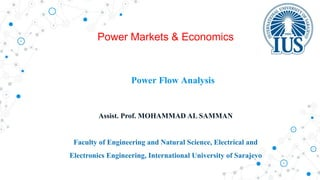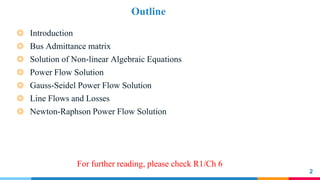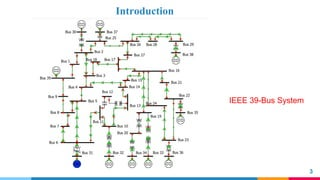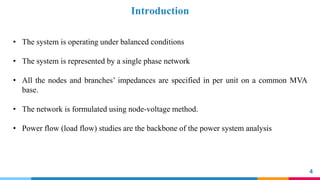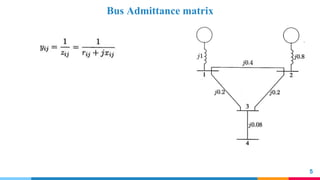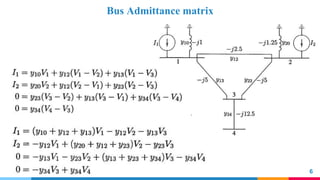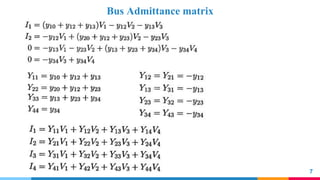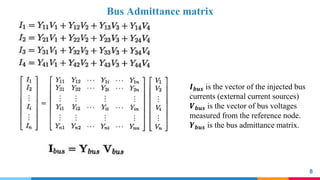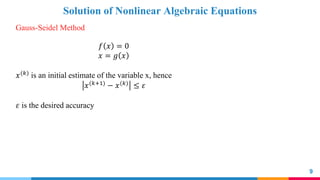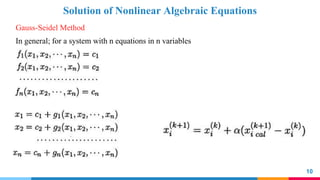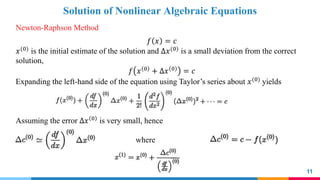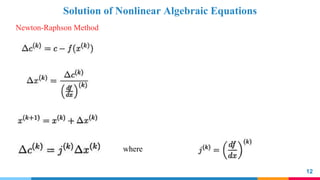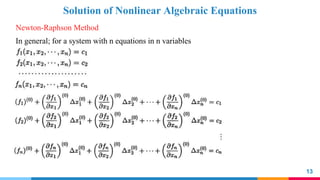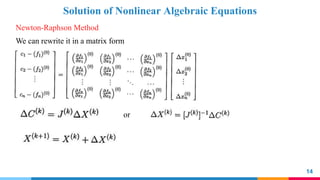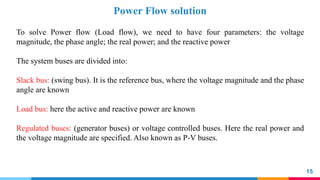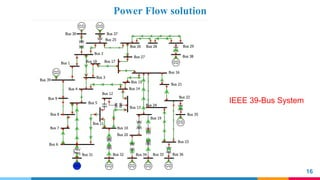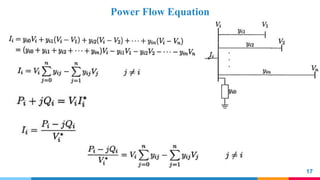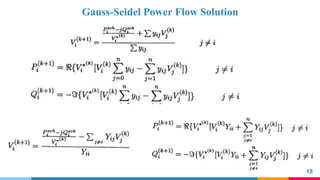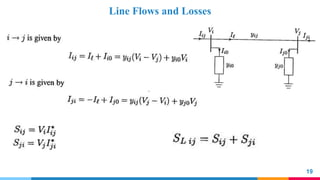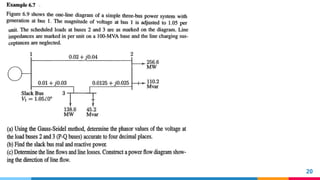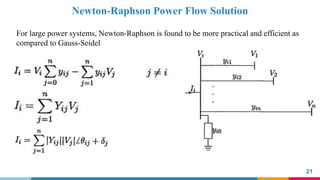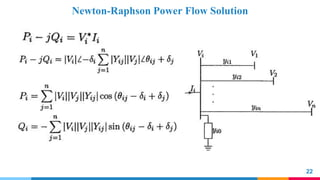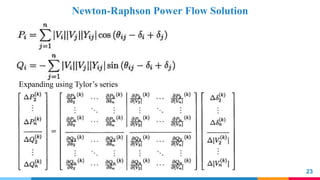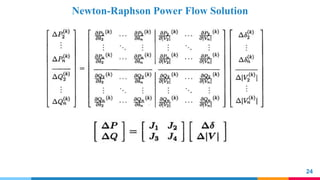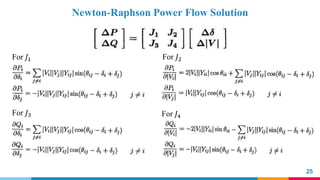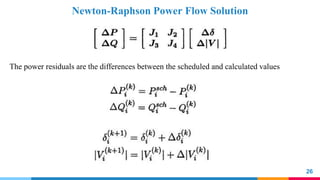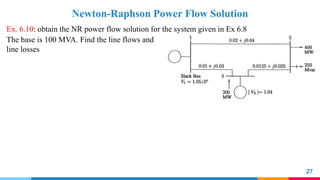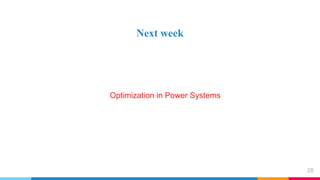1 de 28
Anúncio

### W1_EE566.pptx

1. Power Flow Analysis Assist. Prof. MOHAMMAD AL SAMMAN Faculty of Engineering and Natural Science, Electrical and Electronics Engineering, International University of Sarajevo Power Markets & Economics
2. Outline 2 ◎ Introduction ◎ Bus Admittance matrix ◎ Solution of Non-linear Algebraic Equations ◎ Power Flow Solution ◎ Gauss-Seidel Power Flow Solution ◎ Line Flows and Losses ◎ Newton-Raphson Power Flow Solution For further reading, please check R1/Ch 6
3. Introduction 3 IEEE 39-Bus System
4. Introduction 4 • The system is operating under balanced conditions • The system is represented by a single phase network • All the nodes and branches’ impedances are specified in per unit on a common MVA base. • The network is formulated using node-voltage method. • Power flow (load flow) studies are the backbone of the power system analysis
8. Bus Admittance matrix 8 𝑰𝒃𝒖𝒔 is the vector of the injected bus currents (external current sources) 𝑽𝒃𝒖𝒔 is the vector of bus voltages measured from the reference node. 𝒀𝒃𝒖𝒔 is the bus admittance matrix.
9. Solution of Nonlinear Algebraic Equations 9 Gauss-Seidel Method 𝑓 𝑥 = 0 𝑥 = 𝑔 𝑥 𝑥(𝑘) is an initial estimate of the variable x, hence 𝑥(𝑘+1) − 𝑥(𝑘) ≤ 𝜀 𝜀 is the desired accuracy
10. Solution of Nonlinear Algebraic Equations 10 In general; for a system with n equations in n variables Gauss-Seidel Method
11. Solution of Nonlinear Algebraic Equations 11 𝑓 𝑥 = 𝑐 𝑥(0) is the initial estimate of the solution and ∆𝑥(0) is a small deviation from the correct solution, 𝑓 𝑥 0 + ∆𝑥 0 = 𝑐 Expanding the left-hand side of the equation using Taylor’s series about 𝑥(0) yields Assuming the error ∆𝑥 0 is very small, hence where Newton-Raphson Method
12. Solution of Nonlinear Algebraic Equations 12 Newton-Raphson Method where
13. Solution of Nonlinear Algebraic Equations 13 Newton-Raphson Method In general; for a system with n equations in n variables
14. Solution of Nonlinear Algebraic Equations 14 Newton-Raphson Method We can rewrite it in a matrix form or
15. Power Flow solution 15 To solve Power flow (Load flow), we need to have four parameters: the voltage magnitude, the phase angle; the real power; and the reactive power The system buses are divided into: Slack bus: (swing bus). It is the reference bus, where the voltage magnitude and the phase angle are known Load bus: here the active and reactive power are known Regulated buses: (generator buses) or voltage controlled buses. Here the real power and the voltage magnitude are specified. Also known as P-V buses.
16. Power Flow solution 16 IEEE 39-Bus System
17. Power Flow Equation 17
18. Gauss-Seidel Power Flow Solution 18
19. Line Flows and Losses 19
20. 20
21. Newton-Raphson Power Flow Solution 21 For large power systems, Newton-Raphson is found to be more practical and efficient as compared to Gauss-Seidel
22. Newton-Raphson Power Flow Solution 22
23. Newton-Raphson Power Flow Solution 23 Expanding using Tylor’s series
24. Newton-Raphson Power Flow Solution 24
25. Newton-Raphson Power Flow Solution 25 For 𝐽1 For 𝐽2 For 𝐽3 For 𝐽4
26. Newton-Raphson Power Flow Solution 26 The power residuals are the differences between the scheduled and calculated values
27. Newton-Raphson Power Flow Solution 27 Ex. 6.10: obtain the NR power flow solution for the system given in Ex 6.8 The base is 100 MVA. Find the line flows and line losses
28. Next week 28 Optimization in Power Systems
Anúncio